Open In App
Related Articles
• NCERT Solutions for Class 9 Maths

# Class 9 NCERT Solutions- Chapter 9 Areas of Parallelograms And Triangles – Exercise 9.4

### Question 1. Parallelogram ABCD and rectangle ABEF are on the same base AB and have equal areas. Show that the perimeter of the parallelogram is greater than that of the rectangle.

Solution:

Given: ||gm ABCD and rectangle ABEF lie on the same base AB.

ar(ABCD)=a(ABEF)

to prove: Perimeter of ||gm ABCD> perimeter of rectangle ABEF

AB+BC+CD+DA>AB+BE+EF+FA

Proof:

AB=CD            —-[opposite sides of ||gm and rectangle are equal]

AB=EF             —-[opposite sides of ||gm and rectangle are equal]

→CD=EF

CD+AB=EF+AB        ———1

Here  BC>BF           ———[hypotenuse is always greater than other sides]

AD>AF                   ———[hypotenuse is always greater than other sides]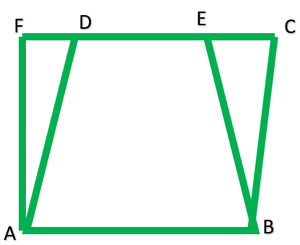### Question 2. In Fig., D and E are two points on BC such that BD = DE = EC. Show that ar (ABD) = ar (ADE) = ar (AEC).

Solution:

Given: In ∆ABC,D and F are two points on BC such that BD=DE=EC

To prove:

Construction: Draw AM ⊥ BC

ar.(ABD)=1/2BD*AM           ———1

=1/2*BD*AM           ——-2

Ar.(AEC)=1/2*EC*MA

=1/2*BD*AM           ————3

From 1 ,2 and 3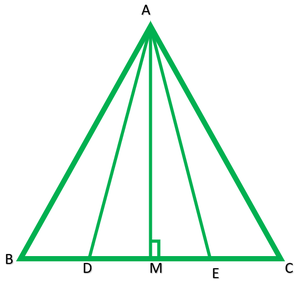### Question 3. In Figure, ABCD, DCFE and ABFE are parallelograms. Show that ar (ADE) = ar (BCF)

Solution:

Given: ||gm ABCD, DCFE, and ABFE

AD = BC               ——[opposite sides of ||gm ABCD]

DE = CF               ——[opposite sides of ||gm DCFE]

AE = BF               ——[opposite sides of ||gm ABFE]

→ar.(ADE) = ar.(BCF)   ——-[congruent triangles have equal in area]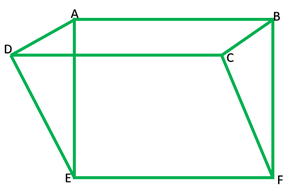### Question 4. In Fig., ABCD is a parallelogram and BC is produced to a point Q such that AD = CQ. If AQ intersects DC at P, show that ar (BPC) = ar (DPQ). [Hint: Join AC.]

Solution:

Given: In fig. ABCD is a ||gm AD=CQ

To prove: ar.(BPC)=ar.()

Construction: Join AC.

In ∆APC and ∆QPD

AC=QD         [opposite sides of ||gm]

∠ 3=∠ 4            [altitude interior angles]

∴∆APC≅∆QPD                      [A.S.A congruence]

→ar.(APC)=ar.(BPC)      [because both lie on the same base PC and between same ||lines PC and AB]

From 1 and 2

ar.(BPC)=ar.(DPQ)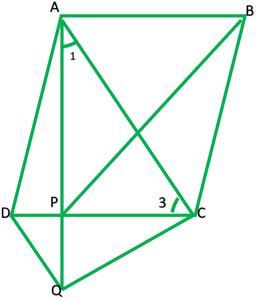### Question 5. In Fig., ABC and BDE are two equilateral triangles such that D is the mid-point of BC. If AE intersects BC at F, show that

(i) ar (BDE) = 14 ar (ABC)

(ii) ar (BDE) = 12 ar (BAE)

(iii) ar (ABC) = 2 ar (BEC)

(iv) ar (BFE) = ar (AFD)

(v) ar (BFE) = 2 ar (FED)

(vi) ar (FED) = 18 ar (AFC)

[Hint: Join EC and AD. Show that BE || AC and DE || AB, etc.]

Solution:

i) Given: ABC and BDE are equilateral triangles D is mid point of BC i.e. BD=DC

To prove:

Proof: Let AB=x=AC=BC

Then BD=x/2=BE=DE

ar.(ABC)=√3/4×2

ar.(BDE)=√3/4*(2/2)2

=√3/4*x2/4

=1/4*√3/4*x2

ar.(BDE)=1/4 ar.(ABC)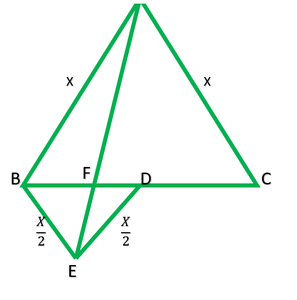ii) to prove; ar.(BDE)=1/2 ar.(BAE)

Construction: Join EC

ED is median of ∆BFC

ar.(BDE)=1/2 ar.(BEC)    ———–1

∠1=∠2=60°             [altitude interior angles]

→BE||AC

AR.(BEC)=ar.(BEA) ————2[both lie on the same base BE and AC between same||lines BE and AC]

Taking 1

ar.(BDE)=1/2 ar.(BAE)     [from 2]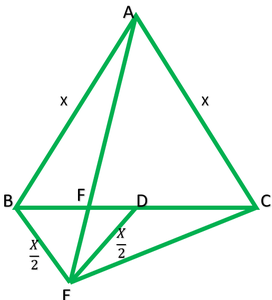iii) To prove: ar.(ABC)=2ar.(BEC)

From i

ar.(BDE)=1/4 ar.(ABC)

from ii

ar.(BDE)=1/2 ar.(BAE)

From I and ii

¼ ar(ABC)=1/2ar.(BAE)

Ar.(ABC)=1/24 ar.(BAE)

=2ar.(BAE)

Ar.(ABC)=2ar.(BFC)     [ar.(BAE)=ar.(BEC) from ii]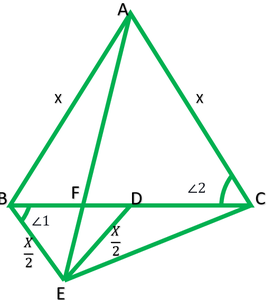iv) To show ar (BFE) = ar (AFD)

Proof: ∠3= ∠4=60°           ——–[angles of equilateral triangles]

Also they are alternate interior angle

∴AE||ED

ar(EDB)=ar(EDA)        ——-[both lie on the same base ED  and Between same || lines ED and AB]

Subtracting ar(EDF) from both sides

ar(EDB)-ar(EDF)=ar.(EDA)-ar.(EDF)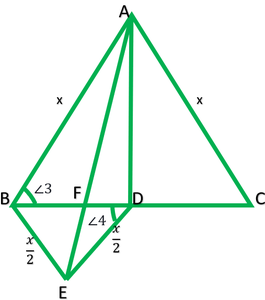v) To show :ar.(BFE)=2ar.(FED)

=x2-(x/2)2

=x2-(x2/4)

=3x2/4

=√3x/4

=1/2*ED*(√3X)/2         ————-1

Construction: Draw EL⊥ BD

→angle is midpoint of BD

In ∆ELD

EL2=ED2-LD2

=(x/2)2-(x/4)2

=x2/4-x2/16

=4x2-x2/16

=3x2/16

EL=√3/10 X2

=√3/4 x

ar(FED)=1/2*FD*EL

=1/2*FD*√3/4 x

=1/2*FD*√3/xx *1/2

ar.(FED)=ar.(AFD)

2ar.(FED)=ar.(BFE)        ——–[in part (iv) we prove ar(AFD=ar.(BFE)]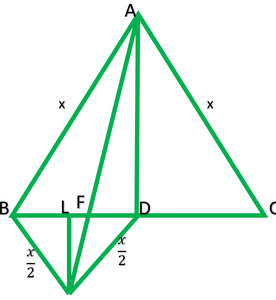(vi) ar.(FED=1/8ar.(AFC))

Solution: ar(BDE)=1/4ar.(ABC)

2ar.(FED)+ar(FED)=1/2ar.(AFC-1/2ar.(AFD))

3ar.(FED)=1/2ar.(AFC-AFD)

3ar.(FED)=1/2ar(AFC)-1/2ar.(AED)

3ar.(FED)=1/2ar.(AFC)-1/2 ar.*2(FED)    ————[FROM (iv) and (v)]

3ar(FED)=1/2 ar.(AFC)-ar.(FED)

3ar.(FED)+ar.(FED)=1/2ar.(AFC)

4ar.(FED)=1/2ar.(AFC)

ar.(FED)=1/2*4 ar.(AFC)

ar.(FED)=1/8 ar.(AFC)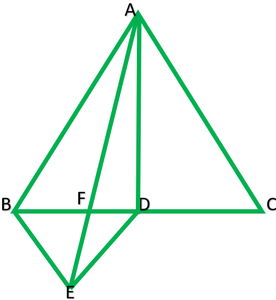### Question 6.Diagonals AC and BD of a quadrilateral ABCD intersect each other at P. Show that ar (APB) × ar (CPD) = ar (APD) × ar (BPC).[Hint :From A and C, draw ⊥s to BD.]

Solution:

Given: ABCD is quadrilateral. Diagonals  AC and BD intersects at P.

To show: ar (APB) × ar (CPD) = ar (APD) × ar (BPC)

Construction: Draw AM ⊥BD &CN ⊥BD

L.H.S.  ar.(APB)*(LPD)

½*PB*AM*1/2*DP*CN      ————-1

¼*PB*AM*DP*LN

R.H.S.  ar.(APD)*ar.(BPC)

½*DP*AM*1/2*BP*CN

¼*DP*AM*BP*CN

¼*PB*AM*DP*CN            ————-2

From 1 and 2

L.H.S=R.H.S

∴ar.(APB)*ar.(CPD)=ar.(APD)*ar.(BPC)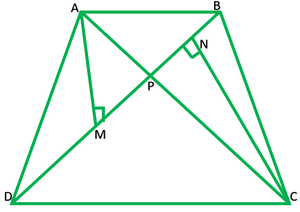### Question 7. P and Q are respectively the mid-points of sides AB and BC of a triangle ABC and R is the mid-point of AP, show that

(i) ar (PRQ) = 12 ar (ARC)

(ii)ar (RQC) = 38 ar (ABC)

(iii) ar (PBQ) = ar (ARC)

Solution:

i) Given: In ∆ABC, P is midpoint of AB, Q is midpoint of BC, R is midpoint of AP

To show:  ar (PRQ) = 12 ar (ARC)

Construction: Join AQ and PC

Solution : ar.(APQ)  ———[∴QR is median of ∆APQ]

=½*1/2ar.(ABQ)   ———[∴QP is median of ∆ABQ]

=1/2*1/2*1/2ar.(ABC)      ———[∴AQ is median of ∆ABC]

=1/8ar.(ABC)     ———-1

ar.(ARC)=1/2(APC)   ——–[∴CR is median of ∆APC]

=1/2*1/2ar.(ABC)     ———-[∴CP is median of ∆ABC]

=1/4ar.(ABC)      ————2

Taking 1

ar.(PRQ)=1/8ar.(ABC)

=1/2*1/4ar.(ABC)   ———-[from 2]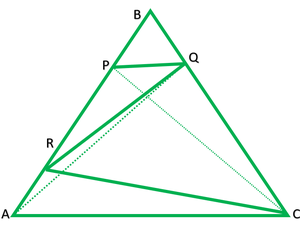ii) ar.(RQC)=3/8ar.(ABC)

solution : ar(ABC)+ar.(ARQ)-ar(ARC)   ——-1

ar(AQC)=1/2(ABC)       [∴AQ is median of ∆ABC]

ar.(ABC)=1/2ar.(APQ)  [∴QR is median of ∆APQ]

=1/2ar.(ABQ) [∴QP is median of ∆ABQ]

=1/2*1/2*1/2ar.(ABC)       [∴AQ is median of ∆ABC]

=1/8ar.(ABC)

ar.(ARC)=1/2ar.(APC)  [∴CR is median of ∆APC]

=1/2ar.(ABC)       [∴CP is median of ∆ABC]

ar.(RQC)=ar.(AQC)+ar.(ARQ)+ar.(ARC)

=1/2ar.(ABC)+1/8ar.(ABC)-1/4ar.(ABC)

=(1/2+1/8-1/4)ar.(ABC)

=(4+1-2/8)ar.(ABC)

=(5-2/8)ar.(ABC)

=3/8ar.(ABC)iii) To show:  ar.(PBQ)=ar.(ARC)

ar(PBQ)=1/2ar.(ABQ)         [∴∆ABQ , QP is median]

=1/2*1/2ar.(ABC)              [∴∆ABC , AQ is median]

=1/4ar.(ABC)         ———1

ar.(ARC)=1/2ar.(APC)    [∴∆APC , CR is median]

=1/2*1/2ar.(ABC)     [∴∆ABC , CP is median]

=1/4ar.(ABC) ——–2

Form I and 2

ar.(PBQ)=ar.(ARC)### Show that:

∆MBC ≅∆ABD

(ii)ar (BYXD) = 2 ar (MBC)

(iii) ar (BYXD) = ar (ABMN)(iv)∆FCB ≅∆ACE

(v) ar (CYXE) = 2 ar (FCB)

(vi)ar (CYXE) = ar (ACFG)

(vii) ar (BCED) = ar (ABMN) + ar (ACFG)

Note: Result (vii) is the famous Theorem of Pythagoras. You shall learn a simpler proof of this theorem in Class X.

Solution:

Given: ∆ABC, right angled at A. BCED,ACFG,ABMN are squares at Y.

i). to prove: ∆MBC ≅∆ABD

solution : In ∆MBC       and    ∆ABD

MB=AB        [∴ABMN is an square ]

BC=BD        [∴BCED is an square]

∠MBC=∠ABD     [∠MBA=∠CBO=90°

∠MBA= +∠CBD+∠ABC

∴∠MBC=∠ABD]

∆MBC≅∆ABD            [S.A.S congruency rule]ii) To show: ar.(BYXD)=2ar.(MBC)

ar.(ABD)=1/2ar.(BYXD)       [Both on the same base BD and between same || lines BD and AX]

ar.(MBC)=1/2ar.(BYXD)       [in (i) ∆MBC≅∆ABD       ∴ar.(MBC)=ar.(ABD)  ]

2ar.(ABC)=ar.( BYXD)

ar.(MBC)=1/2ar.(ABMN)[ ∆MBC and ||gm ABMN  both lie on the same base MB and between same || lines MB and NC.]

2ar.(MBC)=ar.(ABMN)              ————–1

Also,

2ar.(MBC)=ar.(BYXD)              [from ii] —————2

From 1 and 2

ar.(BYXD)-ar.(ABMN)(iv) To show: ∆FCB≅∆ACE

In ∆FCB  and ∆ACE

FC=AC                 [sides of square ACFG]

BC=CE                [sides of square BCED]

∠FCB=∠ACE         [∠ACE=∠BCE=90°

∠ACF+∠ACB=∠BCE+∠ACB

∴∠FCB=∠ACE]

∴∆FCB≅∆ACE        [by S.A.S    congruence ](v) To show : ar.(YXE)=2ar.(FCB)

ar.(ACE)=1/2ar.(CYXE)                 [∆ ACE and ||gm CYXE both lie on the same base CE and between same|| lines CE and AX]

ar. (FCB)=1/2(CYXE)          [in (iv) ∆FCB≅∆ACE               ∴ar.(FCB=ar.(ACE)]

2ar.(FCB)=ar.(CYXE)(vi) To show : ar.(CYXE)=ar.(ACFG)[∆FCB and ||gm ACEFG both lie on the same base CF and BG Between  CF and BG]

2 ar.(FCB)=ar.(ACFG)  ———1

2ar.(FCB)=ar.(CYXE)

From 1 and 2

ar.(CYXE)=ar.(ACFG)

ar.(ABMN)+ar.(ACEFG)

ar.(BYXD)=ar.(ABMN)          [form (iii)]        ———1

ar.(CYXE)=ar.(ACFG)             [From (iv)] ———–2

ar.(BYXD)+ar. (CYXE)=ar.(ABMN)+ar.(ACEFG)(vii) ar.(ABMN)+ar.(ACEFG)

ar.(BYXD)=ar.(ABMN)          [form (iii)]        ———1

ar.(CYXE)=ar.(ACFG)             [From (iv)] ———–2